# NeuReport .pdf

### File information

This PDF 1.5 document has been generated by TeX / MiKTeX pdfTeX-1.40.12, and has been sent on pdf-archive.com on 14/01/2013 at 23:56, from IP address 82.14.x.x. The current document download page has been viewed 985 times.
File size: 667.64 KB (13 pages).
Privacy: public file

### Document preview

Measurement of Mass-Squared Difference (∆m2) of Atmospheric Neutrinos
from Super-Kamiokande
Liam Dodd
December 14, 2012
Abstract
Using data from the Super-Kamiokanda collaboration a difference in the count of muon neutrino events from the
Monte Carlo prediction was observed, and used to show neutrino oscillation. This data was use to calculate the
mass-difference squared ∆m2 between the neutrino flavours as 2.547 × 10−3 eV2 with a 97.3% c.l. of
1.986 × 10−3 eV2 ≤ ∆m2 ≤ 3.565 × 10−3 eV2 . Compared to the accepted value of
−3
2
2.07 × 10 eV &lt; ∆m2 &lt; 2.75 × 10−3 eV2 it was shown that the value for ∆m2 calculated from the data was within the
accepted range The 97.3% c.l. was seen to be larger than the accepted range which inferred an assumption in the
calculation of χ2 simplified the calculation too heavily.

1
1.1

Introduction
This experiment was later improved through the uses
of cadmium chloride, a neutron absorber. When the
cadmium absorbed the neutron it would form an isotope
of cadmium that was unstable and emit a gamma ray to

History of Neutrinos

The neutrino was first hypothesised by Wolfgang Pauli as
n + 108 Cd → 109m Cd → 109 Cd + γ
(3)
a way to explain how energy, momentum and angular momentum were conserved in beta decay. Enrico Fermi later This addition added another means to show this interaction
unified the neutrino, the positron and neutron-proton model was caused by neutrinos, as the gamma ray emitted from the
to give a solid theoretical basis to model of beta decay
cadmium will be detected by the scintillator 5 microseconds
after the pair from the positron annhilation. This relation
n0 → p+ + e− + ν¯e .
(1) of both events is a unique signature of the interaction of
an antineutrino. This discovery eventual lead to Frederick
The neutrino was first detected in 1956, via beta-capture in Reines being awarded the Nobel Prize in 1995.
the Cowan-Reines neutrino experiment. A nuclear reactor
was used as a source of neutrinos with a flux of 5 × 1013
neutrinos per second per square centimeter. This flux was
far higher than what was obtainable from other radioactive 1.2 Neutrino Oscillations
sources. The neutrinos were then predicted to interract with
protons through beta-capture
In 1962 interaction of a muon neutrino were detected2 by
Lederman, Schwartz, and Steinberger. This showed concluThe protons in this experiment were in the form of the sively that neutrinos came in more than one flavour. The
hydrogens within a water molcule. The majority of hy- discovery of the tau lepton in 1975 lead to the expectation
drogens within water have a single proton for a nucleus of a third neutrino flavour existing. The tau neutrino was
which makes the interaction mechanics with neutrinos less only directly detected in 2000 at the DONUT collaboration
complicated1 . The positron emitted from the beta-capture at Fermilab, but its existence had already been inferred
quickly annhilates with an electron and produces a pair using data from the Large Electron-Positron Collider.
of gamma rays. The gamma rays were detected using Observations from LEP of missing energy and momentum
a scintillator, which gives off flashes of light in response in tau decays were theoretically consistent.
to the gamma rays. These light flashes were detected by
Neutrinos were a useful tool in understanding the Sun, as
photomultiplier tubes.
neutrinos pass out of the core unimpeded (2.3 seconds)
1 Single proton nuclei bound within can be modelled by considering
unlike photons which take thousands of years to travel
ν¯e + p+ → n0 + e+ .

(2)

the protons as free, but for heavier nuclei this description is not always
acurate

2 The

1

particle had been theorised in the 1940’s

through the Sun. By observing the neutrinos emitted from
the Sun we are able to get an understanding of what is
going on in the core, and a better measurement of the size.
Measurements of the number of electron neutrinos found a
discrepency as only a third of the expected number were
being measured. A solution to this problem existed in
the form of neutrino oscillations, originally suggested by
Bruno Pontecorvo, in an anolgous form to kaon oscillations.
Neutrino oscillation hypothesises that neutrinos can switch
between flavours as they propogate.

neutrino, and a muon neutrino. A simplification of the
process can be shown as
γ + p, He, Fe... → π + →µ+ + νµ
+

+

µ → e + νe + ν¯µ .

(5)
(6)

This process gives a flux of 2 muon neutrinos for every electron neutrino. Detailed calculations modify this to include
the kaons in the hadronic shower, and the presence of high
energy muons which do not decay in flight before colliding
with the Earth as Lorenzian time dilation allows them to
survive the whole journey.

Neutrino oscillations had a major flaw as the neutrinos must have mass to undergo oscillations. The Standard
Model of particle physics had assumed the neutrinos to be
massless, but neutrino oscillations required a mass difference (∆m) between the three flavours. Super-Kamiokande
and Sudbury Neutrino Observatory have observed neutrino
oscillations in atompsheric and solar neutrinos, respectively.
While we have not measured the mass of each flavour of
neutrino we can produce a mass difference for each of
the oscillations.
This mass difference appears in the
approximation for the survivability of muon neutrinos

1.4

Super-Kamiokande Experiment

The Super-Kamiokande (Super-K) detector was built as
a successor to the KamiokaNDE (Kamiokande Nucleon
Decay Experiment) detector.
which was completed
in 1983 to detect whether proton decay exists. This
experiment was successful in detecting supernova neutrinos,
and was able to directly demonstrate for the first time
2
∆m L
(4) that the Sun was a source of neutrinos. However, the
P (νµ → νµ ) = 1 − sin2 2θ sin2
4E
experiment was unsuccesful in its primary goal of detecting
where θ is the mixing angle among neutrinos, L is the proton decay. The Super-K was built to be a more sensitive
detector, to improve the statistical confidence in the results.
distance travelled by the neutrons, and E is their energy.
The Super-K was the largest water Chrenknov detector and it began operating in 1996. The detector consists
of a stainless steel tank, 39m in diameter and 42m tall,
filled with 50,000 tonnes of ultra pure water and 13,000
photomultiplier detectors3 . The detector is seperated into
two sections, an inner and outer detector. The inner
detector is used for physical studies, while the outer
detector is used to detect charged particles entering the
central volume and to shield the detector from neutrons
produced in the surrounding rocks. The detector is located
1km undergound, within the Mozumi Mine in Japan’s Gifu
Prefecture.
The Super-K collaboration is most famous for its announcement in 1998 of the first evidence of neutrino oscillations,
showing that neutrinos have a non-zero mass, an idea that
has been suggested by theorists before.

Massive neutrinos lead to interesting results within
particle physics. Among them is prediction of sterile
neutrinos due to the helicity of the neutrinos. For massless
spin- 21 particles the helicity is proportional to the chirality
operator. This gives neutrinos as left-handed, and antineutrinos as right-handed, which is seen in observations.
Oscillations require neutrinos to be massive so they must
also have small right and left-handed part (for neutrinos
and anti-neutrinos respectively). As these parts are not
observed, it lead to the hypothesis of sterile neutrinos
existing which carry this unobserved part. These sterile
neutrinos have been suggested as a candidate for dark
matter, as they are predicted to only interact with the
gravitational force. The existence of sterile neutrinos has
gained more support recently as various anomalies within
neutrino experiments offer a greater possibility for these
neutrinos to fit within the accepted theoretical framework
of neutrinos.

Over the lifetime of Super-K it has gone through several upgrades to improve sensitivity and to make repairs. In
2001 6000 photomultipliers imploded, in a form of cascade
failure, so the remaining photomultipliers were repositioned
to create an even distribution, and a protective layer of
1.3 Atmospheric Neutrinos
acrylic was added to the photomultipliers to prevent further
damages. The detctor was restored to full capabilities, and
Neutrinos are produced in the atmosphere from the colli- the electronics were upgrading, allowing Super-K collect
sions of primary cosmic waves with nuclei in the upper at- more data on various natural sources and to act as the far
mosphere. These collisions produce a shower of hadrons, detector for the Tokai-to-Kamioka experiment.
3 Compared to Kamiokande’s 16m diameter, 3000 tonnes of pure
mostly pions. These pions further decay into a muon and a
muon neutrino. The muons decay to an electron, electron water and 3000 photomultipliers
2

Figure 2: A 603 MeV muon event with a well-defined ring.

Figure 1: Schematic Diagram of Super-Kamiokande’s Inner
and Outer Detectors

1.5

Detection of
Kamiokande

Neutrinos

at

Super-

The Super-K detector is a Cherenkov detector. This form of
detector uses the Cherenkov light produced when charged
particles move through a medium faster than the speed of Figure 3: A 492 MeV electron event with a diffuse ring
light in that medium. The charged leptons produced from
the neutrino interactions are typically with suffecient to
Method
exceed the speed of light in the medium, so produce an 2
optical shockwave, known as Cherenkov radiation. These
shockwaves result in a characteristic ring-like pattern that
can be detected by an array of photomultipliers. These The objective of the experiment is to find a value for the
rings can be used to infer direction, energy, and in the case mass difference squared (∆m2 ), and to do this requires us
to perform a simplified χ2 -analysis.
of Super-K, the flavour of the neutrino.
Two flavours of neutrinos were being investigated at
Super-K, and the pattern of the rings could be used to
distinguish the flavours. The muon neutrino events produce
a muon which yields Cherenknov radiation that has a
well-defined circular ring. The electron neutrino events
produce an electron shower which yields a more diffuse ring
in the detector array. A further method for confirming the
flavours is to use the emitted electron from the stopped
muon. In most cases the muon produced will decay into an
electron at a predictable time period later. This electron
will give a second ring at the expected time which can be
used to confirm the original neutrino was a muon neutrino.

2.1

Obtaining Data

This first requires values for the Monte Carlo predictions
and the experimental count to be obtained. The values
can be gathered from figures published in  by finding a
length equivalent to the scale and measuring the points off
of the graph.
The error in the observed values was the variance,
which equated to the square root of the values. The
3

where ∆z is in the error in the result, z is the result, ∆x
and ∆y are the errors in the events, and x and y are the
event counts.

error can be measured directly from the figures but the
count can be measured more accurately (as the length is
larger) than the error itself can be directly. Once all the
data was obtained the six graphs could be reproduced in
Mathematica.

2.2

The expected ratio Rexp of the number of events expected under the assumption of neutrino oscillations, over
the number expected without oscillations, as a function of
∆m2 .
R E2
dEE −γ P (νµ → νµ)
i
(12)
Rexp
= E1 R E2
dEE −γ
E1

Energy and Zenith Angle Distributions

To find ∆m2 we need to model the muon-neutrino fluxes
as a function of length and energy. The primary step is to where P is from Equation 4, and E1 and E2 are the bounds
model the Θ-dependence. The length from the neutrino’s of the energy ranges.
production to it’s detection can be treated as a funtion of
the Zenith angle, L(Θ). For positive values of the Zenith With this χ2 can finally be calculated as
angle the length is the distance from the detector to the
30
i
i
X
atmosphere, and is calculated as
(Rexp
− Robs
)2
χ2 =
.
(13)
σi2
i=1
X0
h0
ln
(7)
Lair '
cos Θ ΛN cos Θ
This approach explicitly neglects all systematic errors,
where h0 , X0 , and ΛN are constants. For Zenith angle values and assumes no correlation between different errors being
2
below zero the neutrinos are passing through the Earth so present. This gives a function in terms of ∆m only. The
2
most probable ∆m value can be found by minimising the
the length is calculated as
χ2 . Four plots of χ2 can be produced, three from each of the
L = Lair − 2REarth cos Θ.
(8) energy ranges, and one of all three ranges summed together.
From these final plots take the ∆m2 value of the high-energy
To look at the energy dependence of the count requires a muon neutrinos and from all three ranges summed together.
sophisticated approach which is simplified for our analysis.
The 99.73% confidence level best-fit range needs to
The number of expected events is modeled as a function
be identified to compare the obtained value with the
Nexp (E) ∝ E −γ .
(9) current accepted value. These can be identified as the
set of values of ∆m2 for which ∆χ2 &lt; 9. On the plot of
The value for γ depends on the energy range as
∆χ2 three horizontal intersecting lines are drawn across
 5
the graph at ∆χ2 + 1, 4, 9 for the ∆χ2 value at the local
E &gt; 1.33GeV
 2
minimum. These lines represent various confidence levels,
2 0.4GeV &lt; E &lt; 1.33GeV
γ=
(10) but the focus of this experiment looks at the values of ∆m2
 4
0.1GeV &lt; E &lt; 0.4GeV
3
when the lines at ∆χ2 + 9 intersect. The two localised
intersection values are the 99.73% c.l. of our ∆m2 value.
Data from  can be obtained for the experimental neutrino
flux values at Kamiokande. These values can be compared
Using the two values of ∆m2 obtained from the two
against the simplistic function to see how good an approxiplots and substituting them into Equation 12 to find
mation the function is.
numerical values for Rexp . These are then plotted against
the Robs and it is now possible to qualitatively check which
value of ∆m2 fits best to the observed data.

2.3

Muon-Neutrino Analysis

i
For the final part a table of the 30 values of Robs
must
i
be produced, where Robs is defined as the ratio of detected
events over Monte Carlo expected events. For each of these
values an error σi needs to be obtained, using the error
propagation formula
s
2 
2
∆x
∆y
∆z = z ·
+
(11)
x
y

4

3

Results

3.1

Super-Kamiokande Data

Figure 6: Sub-GeV e-like, P≥400MeV/c

Figure 4: Sub-GeV e-like, P≤400MeV/c

Figure 7: Sub-GeV µ-like, P≥400MeV/c

Figure 5: Sub-GeV µ-like, P≤400MeV/c
.

5

Figure 8: Multi-GeV e-like
Figure 10: Plot of L(Θ)

Figure 9: Multi-GeV µ-like + PC

3.2

Energy Distributions
Figure 11: Plot of E −γ , blue line, against the observed values over the range 0.1GeV &lt; E &lt; 0.4GeV, red line.

.

Figure 12: Plot of E −γ against the observed values over the
range 0.4GeV &lt; E &lt; 1.3GeV

6

Figure 14: A plot of χ2 against ∆m2 for the low energy
Figure 13: Plot of E against the observed values over the range, 0.1GeV &lt; E &lt; 0.4GeV, with χ2 reaching a minimum
range 1.3GeV &lt; E &lt; 3.0GeV
at ∆m2 = 0.00368907
−γ

3.3

χ2 Calculation

.

Figure 15: A plot of χ2 against ∆m2 for the low energy
range, 0.4GeV &lt; E &lt; 1.3GeV, with χ2 reaching a minimum
at ∆m2 = 0.00397056

Figure 16: A plot of χ2 against ∆m2 for the high energy range, 1.3GeV &lt; E, with confidence levels drawn
for ∆χ2 = 1, 4, 9, and with χ2 reaching a minimum at
∆m2 = 0.00254714
7

Figure 17: A plot of χ2 against ∆m2 for the sum of all three
energy ranges, with confidence levels drawn for ∆χ2 = 1, 4, 9
and with χ2 reaching a minimum at ∆m2 = 0.0034

Figure 20: Plot of Rexp , the red plot, against Robs , the blue
plot, for 1.3GeV &lt; E using the ∆m2 value from the high
energy χ2

Figure 18: Plot of Rexp , the red plot, against Robs , the blue
plot, for 0.1GeV &lt; E &lt; 0.4GeV using the ∆m2 value from
the high energy χ2

Figure 21: Plot of Rexp , the green plot, against Robs , the
blue plot, for 0.1GeV &lt; E &lt; 0.4GeV using the ∆m2 value
from the sum of the three energy ranges χ2

Figure 19: Plot of Rexp , the red plot, against Robs , the blue
plot, for 0.4GeV &lt; E &lt; 1.3GeV using the ∆m2 value from
the high energy χ2

Figure 22: Plot of Rexp , the gree plot, against Robs , the blue
plot, for 0.4GeV &lt; E &lt; 1.3GeV using the ∆m2 value from
the sum of the three energy ranges χ2

8

An interesting observation of the data was the peak
of predicted Monte Carlo count at Θ = 0, but not for
the lower energy values. The predictions for low energy
neutrinos are due to the geomagnetic field effects while
the fluxes peaked at the horizontal direction, cos Θ = 0
for higher energy neutrinos are due to the larger decay of
muons in the horizontal direction.
To model the energy dependence a simplified function, Equation 9, was used. This approach is unable to
produce exact predictions of the experimentally observed
results due to the points not matching perfectly along the
points.
Figure 23: Plot of Rexp , the green plot, against Robs , the The piecewise nature of the function also means that
blue plot, for 1.3GeV &lt; E using the ∆m2 value from the at the range boundaries the fit is offset from the observed
points. However, the functions follow the shape of the data
sum of the three energy ranges χ2
points and fits close to the numerical values of the points.
This crude approximation gives values which can be used
for the calculation of χ2 .
4 Discussion and Conclusion

4.1

Evidence of Oscillation from Super- 4.3
Kamiokande Data

χ2 Calculation

Four plots were found for χ2 , three of the energy ranges
The data from Super-K qualatitively appears to deviate and one of all three plots summed. Across these four
from expected results for the µ-like neutrinos at all energy plots four different shapes were seen, though a common
levels, while e-like neutrinos match the predicted Monte feature of approaching a local minimum within the range
Carlo values. To form a quantative argument to show this of 2 × 10−3 eV2 6 ∆m2 6 4 × 10−3 eV2 . The rest of the
the ratio of predicted against observed can be calculated. shapes are independent from another, as each χ2 function
For results that match the prediction the number will be is expressed through different functions4 .
approximately 1, and will be far from 1 for results that
don’t.
Three of the four values obtained are similar to one
another, while the Multi-GeV result is significantly lower
Figures 25 to 30 show the values for these ratios. For at 2.54714 × 10−3 eV2 . The test of these values came
the µ-like events the ratios are far from 1, and for the e-like from substituting the obtained ∆m2 into Equation 12 and
events the ratio is far closer (with some deviation around plotting them against the observed values.
the point which is to be expected due to low count values).
This indicates the flux of muon neutrinos is significantly Figure 18 to 20 show the plots using the Multi-GeV
lower than expected, which leads to the possibility that the ∆m2 . The expected values deviate far from the observed
muons are oscillating to taus which are not detected by values, in the two low energy graphs the expected values lie
Super-K.
within the uncertainty of many points. For the high energy
plot the expected values match with the observed values.
The Multi-GeV ∆m2 provides a good fit for high energy
plot, and satisfactory plots for the lower energies.

4.2

Energy and Zenith Angle Distributions

Figures 21 to 23 show the plots using the ∆m2 from
the χ2 of the three ranges summed. For all three range
Using Equation 8 we produced a table of length values plots the expected values outside of the errors of the
(Figure 24) and plotted them as in Figure 10. The results observed values. This is most distinct in the lower energies
appear sensible as the most negative Zenith angle is a large where the expected values are double the predicted value
fraction of the Earth’s diameter, as is expected of Neutrinos at some angles.
passing ’down’ through the Earth from the atmosphere on
4 Low energy range is a series of Gamma function, mid energy range
the other side.
is composed of sin functions and the high energy from FresnelS functions

9

4.4

Final Value of ∆m2

From the previous analysis the more suitable value of ∆m2 is
2.547×10−3 eV2 . To obtain a value for the 99.73% confidence
level, the 3-σ of the normal distribution must be determined.
The horizontal plot on Figure 16 at ∆χ2 = 9 represents this
confidence level. The values for ∆m2 can be read off for
when χ2 crosses this line. This gives the confidence level
values as
1.986 × 10−3 eV2 ≤ ∆m2 ≤ 3.565 × 10−3 eV2 .

(14)

The current bestfit based on all the neutrino data yields the
∆m2 ’s bounds at 99.73% confidence level as
2.07−3 eV2 &lt; ∆m2 &lt; 2.75−3 eV2

(15)

The final value for ∆m2 from our experiment lies within
this range, but the 99.73% c.l. calculated is larger than the
accepted range. There are a series of possibilities for where
this error can have come from.
One major area may be from our energy distribution
assumption. The value of χ2 as a function of ∆m2 was
calculated through an integral which used the values for γ
from Equation 10, and used the energy ranges postulated
for these values of γ. The assumption used is crude and
lacks some sophistication which may have lead to result for
χ2 lacking some important features.
Small changes can lead to a difference in the shape of
the graph which could narrow down the 3-σ bands to
values closer in magnitude to the accepted value. The
Multi-GeV χ2 plot has hints that it may be narrow under
a more accurate analysis as the minimum takes the form of
a ’double-dip’. If the second minimum was not present the
valley in which the minimum sits would be considerably
narrower.

References
 The reines-cowan experiments. Los Alamos Science, 25,
1997.

 S. Fukuda, Y. Fukuda, T. Hayakawa, E. Ichihara, M. Ishitsuka, Y. Itow, T. Kajita, J. Kameda,
K. Kaneyuki, S. Kasuga, K. Kobayashi, Y. Kobayashi,
Y. Koshio, M. Miura, S. Moriyama, M. Nakahata,
S. Nakayama, T. Namba, Y. Obayashi, A. Okada,
M. Oketa, K. Okumura, T. Oyabu, N. Sakurai, M. Shiozawa, Y. Suzuki, Y. Takeuchi, T. Toshito, Y. Totsuka,
S. Yamada, S. Desai, M. Earl, J.T. Hong, E. Kearns,
M. Masuzawa, M.D. Messier, J.L. Stone, L.R. Sulak,
C.W. Walter, W. Wang, K. Scholberg, T. Barszczak,
D. Casper, D.W. Liu, W. Gajewski, P.G. Halverson,
J. Hsu, W.R. Kropp, S. Mine, L.R. Price, F. Reines,
M. Smy, H.W. Sobel, M.R. Vagins, K.S. Ganezer,
W.E. Keig, R.W. Ellsworth, S. Tasaka, J.W. Flanagan, A. Kibayashi, J.G. Learned, S. Matsuno, V.J.
Stenger, Y. Hayato, T. Ishii, A. Ichikawa, J. Kanzaki,
T. Kobayashi, T. Maruyama, K. Nakamura, Y. Oyama,
A. Sakai, M. Sakuda, O. Sasaki, S. Echigo, T. Iwashita,
M. Kohama, A.T. Suzuki, M. Hasegawa, T. Inagaki,
I. Kato, H. Maesaka, T. Nakaya, K. Nishikawa, S. Yamamoto, T.J. Haines, B.K. Kim, R. Sanford, R. Svoboda, E. Blaufuss, M.L. Chen, Z. Conner, J.A. Goodman, E. Guillian, G.W. Sullivan, D. Turcan, A. Habig,
M. Ackerman, F. Goebel, J. Hill, C.K. Jung, T. Kato,
D. Kerr, M. Malek, K. Martens, C. Mauger, C. McGrew, E. Sharkey, B. Viren, C. Yanagisawa, W. Doki,
S. Inaba, K. Ito, M. Kirisawa, M. Kitaguchi, C. Mitsuda, K. Miyano, C. Saji, M. Takahata, M. Takahashi,
K. Higuchi, Y. Kajiyama, A. Kusano, Y. Nagashima,
K. Nitta, M. Takita, T. Yamaguchi, M. Yoshida,
H.I. Kim, S.B. Kim, J. Yoo, H. Okazawa, M. Etoh,
K. Fujita, Y. Gando, A. Hasegawa, T. Hasegawa,
S. Hatakeyama, K. Inoue, K. Ishihara, T. Iwamoto,
M. Koga, I. Nishiyama, H. Ogawa, J. Shirai, A. Suzuki,
T. Takayama, F. Tsushima, M. Koshiba, Y. Ichikawa,
T. Hashimoto, Y. Hatakeyama, M. Koike, T. Horiuchi, M. Nemoto, K. Nishijima, H. Takeda, H. Fujiyasu, T. Futagami, H. Ishino, Y. Kanaya, M. Morii,
H. Nishihama, H. Nishimura, T. Suzuki, Y. Watanabe,
D. Kielczewska, U. Golebiewska, H.G. Berns, S.B. Boyd,
R.A. Doyle, J.S. George, A.L. Stachyra, L.L. Wai, R.J.
Wilkes, K.K. Young, and H. Kobayashi. The superkamiokande detector. Nuclear Instruments and Methods
in Physics Research Section A: Accelerators, Spectrometers, Detectors and Associated Equipment, 501(23):418
– 462, 2003.

 Subinoy Das, Wick Haxton, Laveder Marco, Manfred
Lindner, Jonathon M. Link, Alexander Merie, Oleg  http://www.ps.uci.edu/tomba/sk/tscan/compare mu e/.
Ruchayskly, and Jian Tang. Light sterile neutrinos: A
white paper arxiv:1204.5379[hep.ph]. 2012.
 J. Kameda. Detailed Studies of Neutrino Oscillation
with Atmospheric Neutrinos of Wide Energy Range from
 M. Honda, T. Kajita, K. Kasahara, and S. Mi100 MeV to 1000 GeV in Super-Kamiokande. PhD thedorikawa. New calculation of the atmospheric neutrino
sis, Ph. D. Thesis, University of Tokyo, 2002.
flux in a three-dimensional scheme. Physical Review D,
70(4):043008, 2004.
 T. K. Gaisser and Todor Stanev. Path length distributions of atmospheric neutrinos. Phys. Rev. D, 57:1977–
e.html.
10

 M. Honda, T. Kajita, K. Kasahara, and S. Midorikawa.
Calculation of the flux of atmospheric neutrinos. Physical Review D, 52(9):4985, 1995.

A

Tables
Zenith Angle (cos Θ)
-0.9
-.047
-0.5
-0.3
-0.1
0.1
0.3
0.5
0.7
0.9

Length L (km)
11496.6
8952.26
6414.49
3898.41
1560.57
284.954
71.5476
36.39
22.9165
16.0369

Figure 24: Values obtained from Equation 8 for L for each
Zenith Angle
i
1
2
3
4
5
6
7
8
9
10

i
Robs
0.607843
0.582781
0.714286
0.555556
0.631944
0.619718
0.645833
0.602649
0.687075
0.671429

σi
0.079923 7
0.0781583
0.0912681
0.0751555
0.0846274
0.0840761
0.0859156
0.0799766
0.0887995
0.0895323

i
Figure 25: Table of Robs
(observed Super-K value, divided
by the Monte Carlo prediction) values and the error in each
value. For the µ-like neutrino events of the energy range
0.1 &lt; E &lt; 0.4

.

11

i
11
12
13
14
15
16
17
18
19
20

i
Robs
0.66055
0.422727
0.509174
0.623853
0.68599
0.705069
0.904306
0.855769
0.917476
0.00481

σi
0.0709334
0.0522853
0.059371
0.0681689
0.0747483
0.0744315
0.0907722
0.0873793
0.0924121
0.0984116

i
1
2
3
4
5
6
7
8
9
10

i
Figure 26: Table of Robs
(observed Super-K value, divided
by the Monte Carlo prediction) values and the error in each
value. For the µ-like neutrino events of the energy range
0.4 &lt; E &lt; 1.3

i
21
22
23
24
25
26
27
28
29
30

i
Robs
0.459459
0.493151
0.435583
0.526596
0.607656
0.769231
0.853107
0.94375
0.920863
0.945946

i
Robs
1.2619
1.22581
0.991597
1.08943
1.10169
1.08696
1.03502
0.894309
1.1453
1.14286

i
Figure 28: Table of Robs
(observed Super-K value, divided
by the Monte Carlo prediction) values and the error in each
value. For the e-like neutrino events of the energy range
0.1 &lt; E &lt; 0.4

σi
0.0673114
0.0710175
0.0619378
0.0653916
0.0683679
0.0808889
0.0945073
0.107075
0.112808
0.111524

i
Figure 27: Table of Robs
(observed Super-K value, divided
by the Monte Carlo prediction) values and the error in each
value. For the µ-like neutrino events of the energy range
1.3 &lt; E

12

i
1
2
3
4
5
6
7
8
9
10

B

i
Robs
0.991736
1.04762
1.11382
0.97619
0.967742
1.02439
1.05932
0.922414
1.15126
0.88785

i
Figure 29: Table of Robs
(observed Super-K value, divided
by the Monte Carlo prediction) values and the error in each
value. For the e-like neutrino events of the energy range
0.4 &lt; E &lt; 1.3

i
1
2
3
4
5
6
7
8
9
10

i
Robs
0.944444
0.955556
0.830508
1.39344
1.07143
0.88
1.01515
1
0.86
1.125

i
Figure 30: Table of Robs
(observed Super-K value, divided
by the Monte Carlo prediction) values and the error in each
value. For the e-like neutrino events of the energy range
1.3 &lt; E

13

Mathematica Script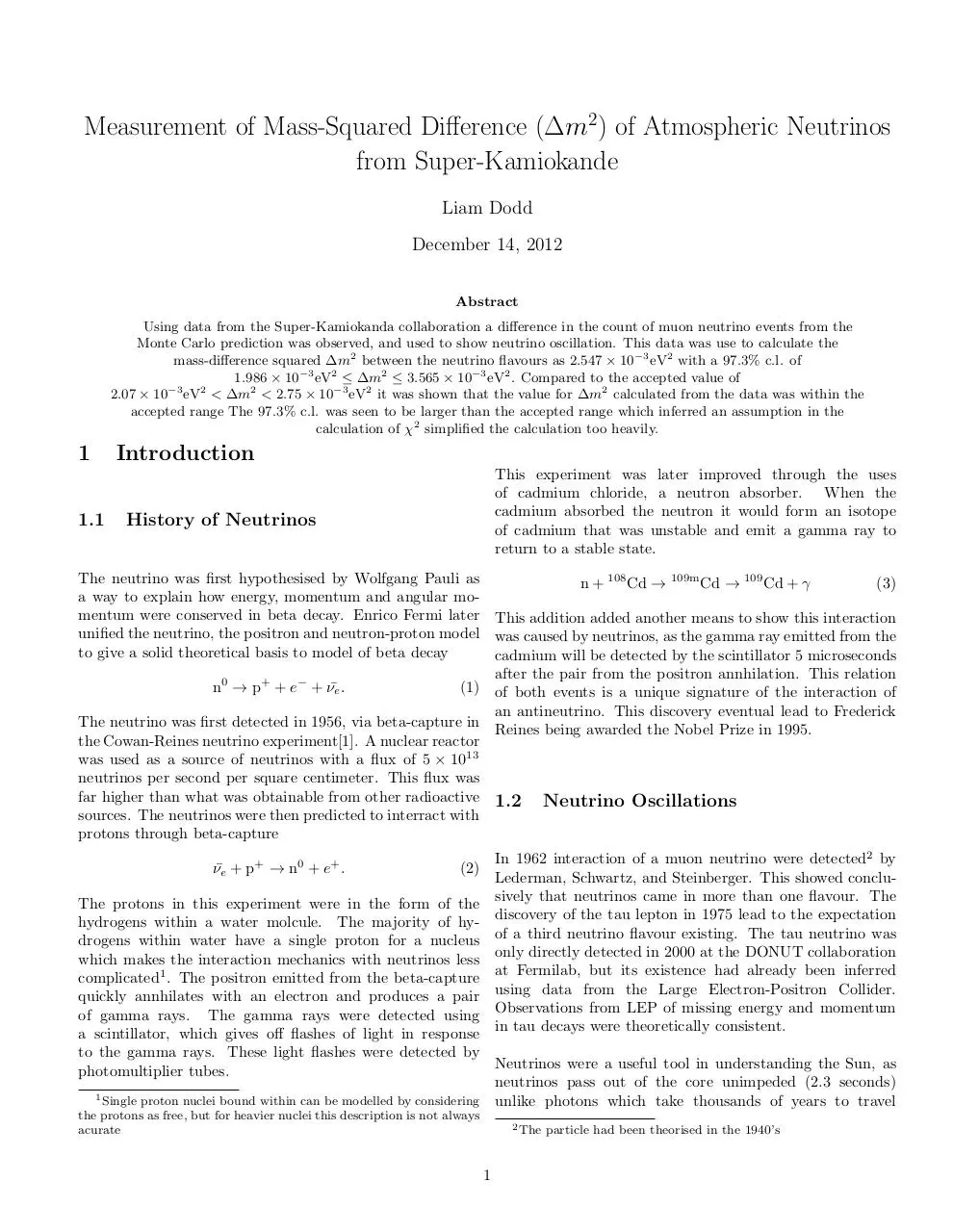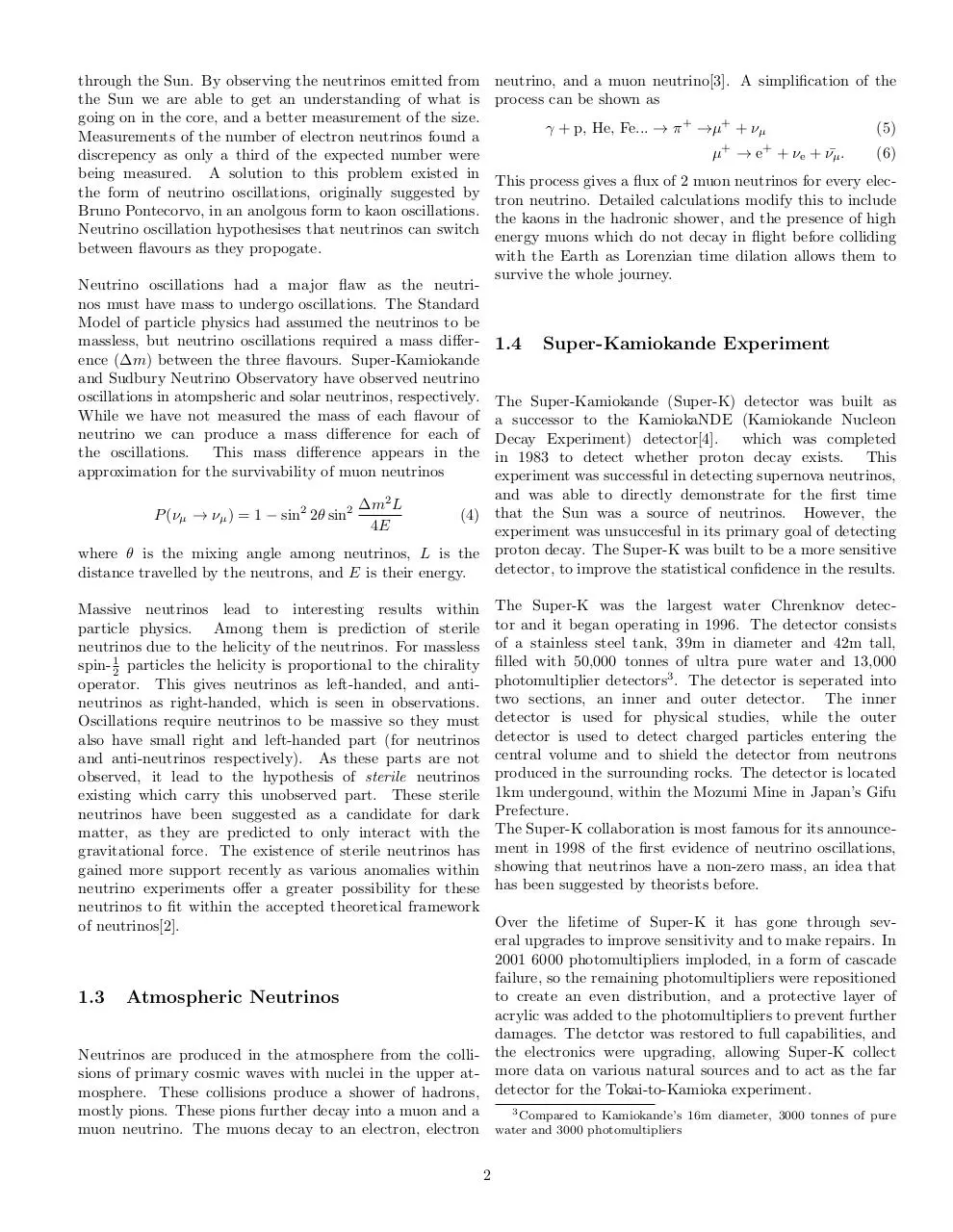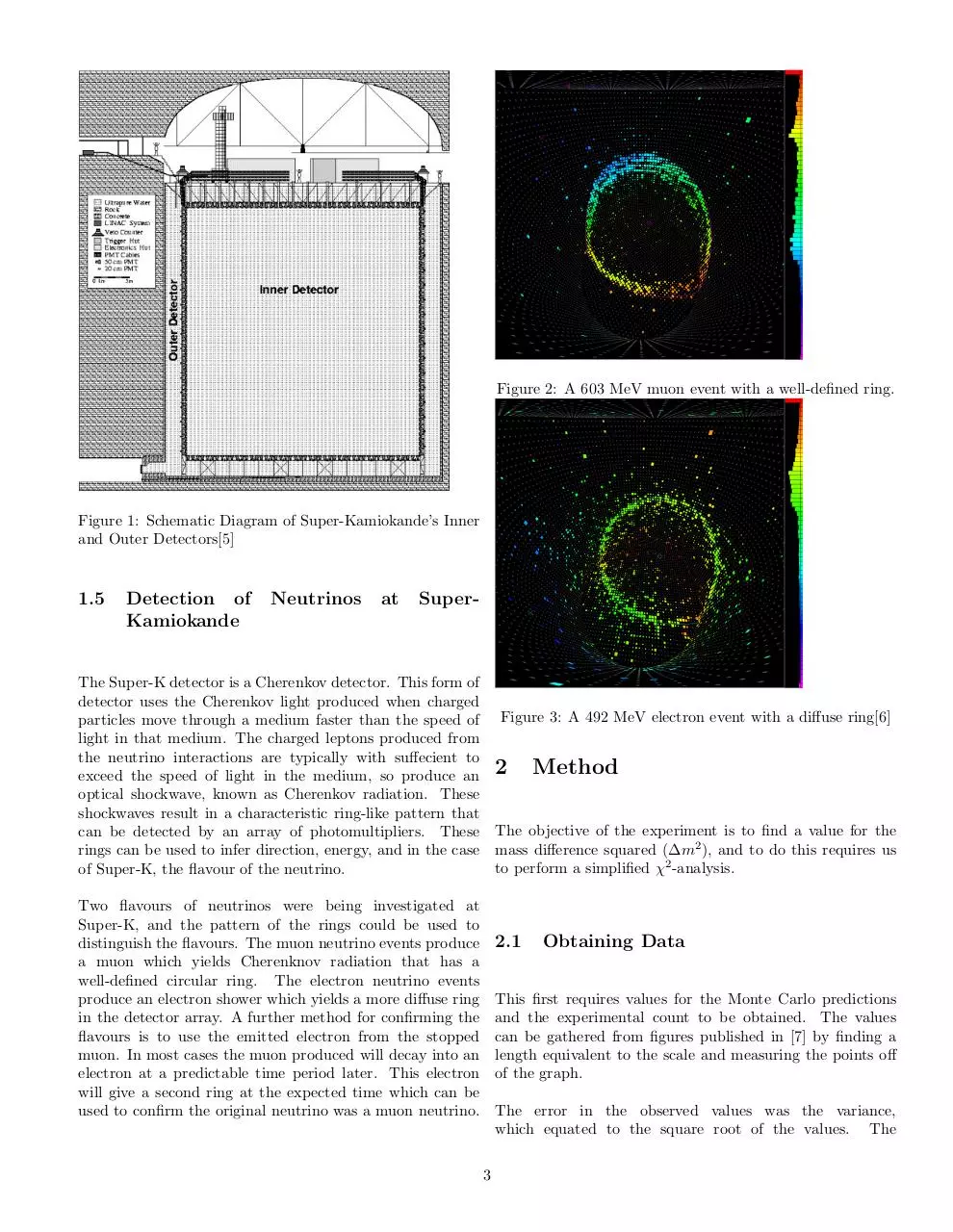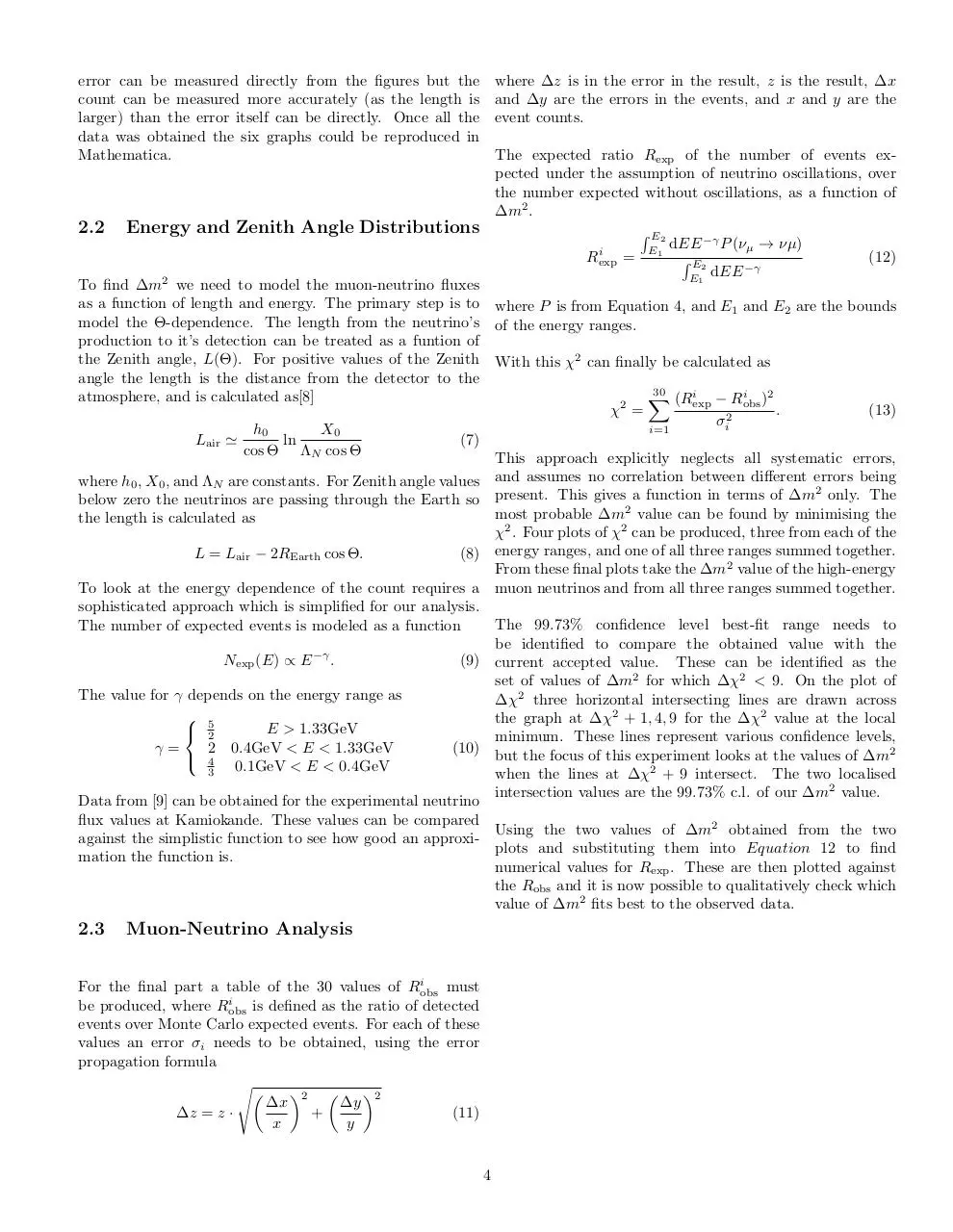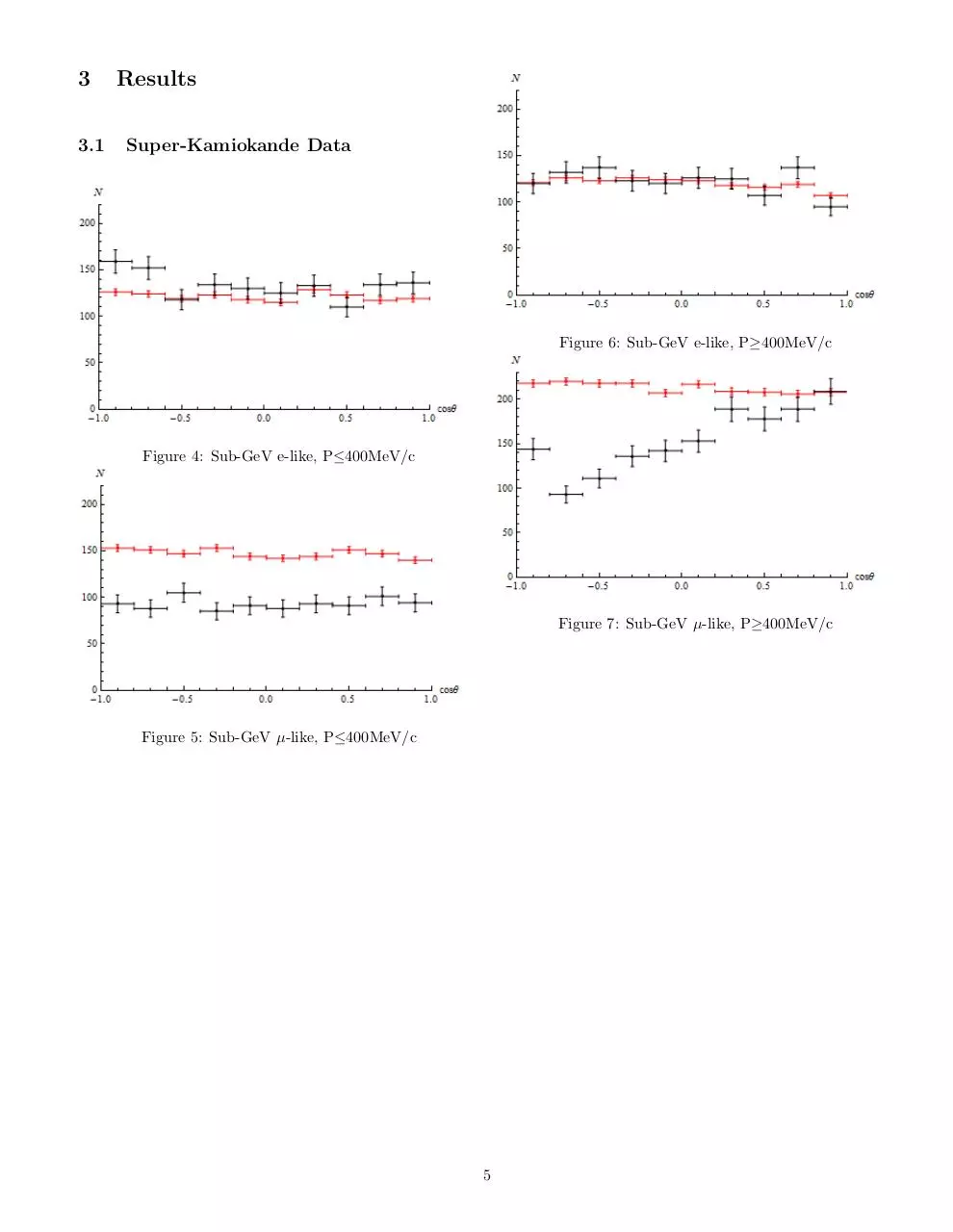NeuReport.pdf (PDF, 667.64 KB)

### Share on social networks

#### HTML Code

Copy the following HTML code to share your document on a Website or Blog